## Journal of Applied Mathematics and Statistical Applications

All submissions of the EM system will be redirected to Online Manuscript Submission System. Authors are requested to submit articles directly to Online Manuscript Submission System of respective journal.

Review Article - Journal of Applied Mathematics and Statistical Applications (2018) Volume 1, Issue 1

## Biharmonic timelike curves according to Bishop frame in Minkowski 4-space

*Corresponding Author:
Solouma EM
Faculty of Science Department of Mathematics Beni-Suef University Beni-Suef Egypt
Tel: +2 (082) 232 48 79
E-mail: [email protected]

Accepted date: November 29, 2017

Citation: Solouma EM, Wageeda MM. Biharmonic timelike curves according to Bishop frame in Minkowski 4-space. J Appl Math Stat App. 2017;1(1):1-3.

### Abstract

In the last decade there has been a growing interest in the theory of biharmonic maps which can be divided in two main research directions. On the one side, constructing the examples and classification results has become important from the differential geometric aspect. The other side is the analytic aspect from the point of view of partial differential equations, because biharmonic maps are solutions of a fourth order strongly elliptic semi linear PDE.Biharmonic curves γ : I ?⊂???→(N, h) of a Riemannian manifold are the solutions of the fourth order differential equation 3 R( , 3 ) 0, γ γ γ γ γ γ ′ ′ ∇ ′ − ′ ∇ ′ ′ = (1) where,∇ is the Levi-Civita connection on (N, h) and R is its curvature operator. As we shall detail in the next section, they arise from a variational problem and are a natural generalization of geodesics. In the last decade biharmonic curves have been extensively studied and classified in several spaces by analytical inspection of Equation 1 [1-15].Although much work has been done, the full understanding of biharmonic curves in a surface of the Euclidean threedimensional space is far from been achieved. As yet, we have a clear picture of biharmonic curves in a surface only in the case that the surface is invariant by the action of a one parameter group of isometries of the ambient space. For example, in the study by Caddeo et al.  it was proved that a biharmonic curve on a surface of revolution in the Euclidean space must be a parallel that is an orbit of the action of the group on the surface. This property was then generalized to invariant surfaces in a 3-dimensional manifold .In this paper, we study biharmonic non-lightlike curves according to Bishop frame in Minkowski 4-space 4 1 E . We give some characterizations for curvatures of a biharmonic non-lightlike curve in 4 1 E . This paper is organized as follows: Section 2 gives some basic concepts of the Frenet frame and Bishop frame of a curve in 4 1 E . Section 3 obtained some characterizations for curvature of these curves with respect to the principal curvature functions ( ) 1ks , ( ) ( ) 2 3 k s , k s according to Bishop frame

### Keywords

Minkowski space-time, Biharmonic curve, Bishop frame, Heisenberg group.

### Introduction

In the last decade there has been a growing interest in the theory of biharmonic maps which can be divided in two main research directions. On the one side, constructing the examples and classification results has become important from the differential geometric aspect. The other side is the analytic aspect from the point of view of partial differential equations, because biharmonic maps are solutions of a fourth order strongly elliptic semi linear PDE.

Biharmonic curvesof a Riemannian manifold are the solutions of the fourth order differential equation(1)

where,∇ is the Levi-Civita connection on (N, h) and R is its curvature operator. As we shall detail in the next section, they arise from a variational problem and are a natural generalization of geodesics. In the last decade biharmonic curves have been extensively studied and classified in several spaces by analytical inspection of Equation 1 [1-15].

Although much work has been done, the full understanding of biharmonic curves in a surface of the Euclidean threedimensional space is far from been achieved. As yet, we have a clear picture of biharmonic curves in a surface only in the case that the surface is invariant by the action of a one parameter group of isometries of the ambient space. For example, in the study by Caddeo et al.  it was proved that a biharmonic curve on a surface of revolution in the Euclidean space must be a parallel that is an orbit of the action of the group on the surface. This property was then generalized to invariant surfaces in a 3-dimensional manifold .

In this paper, we study biharmonic non-lightlike curves according to Bishop frame in Minkowski 4-space E41 . We give some characterizations for curvatures of a biharmonic non-lightlike curve in E41 . This paper is organized as follows: Section 2 gives some basic concepts of the Frenet frame and Bishop frame of a curve in E41 . Section 3 obtained some characterizations for curvature of these curves with respect to the principal curvature functionsaccording to Bishop frame.

### Preliminaries

The Minkowski space-time is four-dimensional Euclidean space provided with the Lorentzian inner product,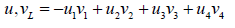where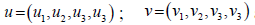are the rectangular coordinate system of E41 . Any vector u in E41 can be characterized as follows: the vector u is called spacelike, lightlike or timelike if ,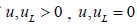or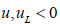respectively.The norm of a vector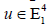is given by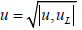.

Any arbitrary curve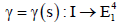is spacelike, timelike or lightlike (null), if all of its velocity vector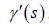are spacelike, timelike or lightlike (null), respectively. If, then γ is called unit speed curve . For any unit speed timelike curve γ with Frenet-Serret frame { T,N,B1 ,B2 }, the Frenet formulas of the curve γ can be given as:where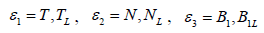and, andandare curvature, first and second torsion functions of the curve γ respectively. Here, we call the value of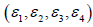as the character of Frenet frame of the curve γ .

The Bishop frame or parallel transport frame is an alternative approach to define a moving frame that is well defined even when the curve has vanished second derivative. We can transport an orthonormal frame along a curve simply by Bishoping each component of the frame [1-3,12,13]. The tangent vector and any convenient arbitrary basis for the remainder of the frame are used. The Bishop frame is expressed as,

whereare principal curvatures of the timelike curve according to the parallel frame and their expression as following: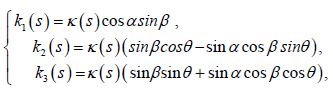andwhere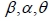are the spherical angle between the spacelike vectors N3 and N3 , spacelike vectors N3 and N3 , spacelike vectors N3 and N3 respectively . We shall call the set {T, N1 ,N2 ,N3} as Bishop frame of γ in the Minkowski 4-spaceγ .

### Discussion and Conclusion

Biharmonic non-lightlike curves according to Bishop frame in E41

In this section we give the notation of biharmonic non-lightlike curves in E41 according to Bishop frame. We also obtain some characterizations for curvature of these curves. Let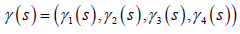be a unit speed timelike curve parametrized by arc-length in E41. The corresponding Bishop frame formula has the following form: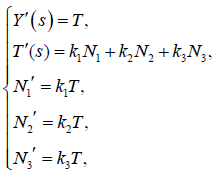(2)

where k2 , k2 and γ are the principal curvature functions according to Bishop frame of the curve γ . Now, the biharmonic equation for the curve γ reduces to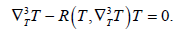(3)

Here, the curve γ is called a biharmonic curve if it is a solution of the Equation (3).

Theorem 3.1: Let γ be a unit speed timelike curve with nonzero principal curvature k1 , k2 and k3 in E41 . Then γ is a biharmonic curve if and only if the following conditions are satisfied:(4)

where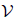is non-zero constant of integration.

Proof. Using the Bishop Equation (2) and biharmonic Equation (3), we obtain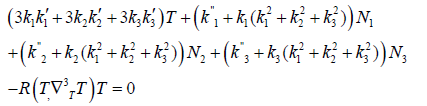(5)

In Minkowski 4-space E41 , the Riemannian curvature is zero, then we have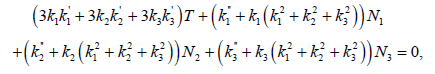(6)

By Equation (6), we see that the curve γ is a unit speed biharmonic curve if and only if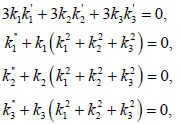(7)

Hence from the Equation (7), we get the result which complete our proof.

As a consequence of the Equation (4) we obtain the following Corollary;

Corollary 3.2: Let γ be a unit speed timelike curve with non-zero principal curvature k1 , k2 and k3 in E41. Then γ is a biharmonic curve if and only if the following conditions are satisfied:(8)

Whereis non-zero constant of integration.

Theorem 3.3: Let γ be a unit speed timelike curve with non-zero principal curvature k1 , k2 and k3 in E41 . Then γ is a biharmonic curve if and only if the following conditions are satisfied: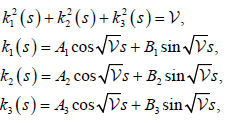(9)

where Ai , Bj for 1≤ i, j ≤ 3 andare constants of integration. Proof. Directly, by using Equation (8), we have Equation (9).

Corollary 3.4: If A1= A2 = A3 and B1 = B2 = B3 , then we have k1(s) = k2(s) = k3(s) .

Corollary 3.5: Let γ be a unit speed timelike biharmonic curve with non-zero principal curvature k1 , k2 and k3 in E41 , thenwhereandAi, Bj for 1≤ i, j ≤ 3 are constants of integration.